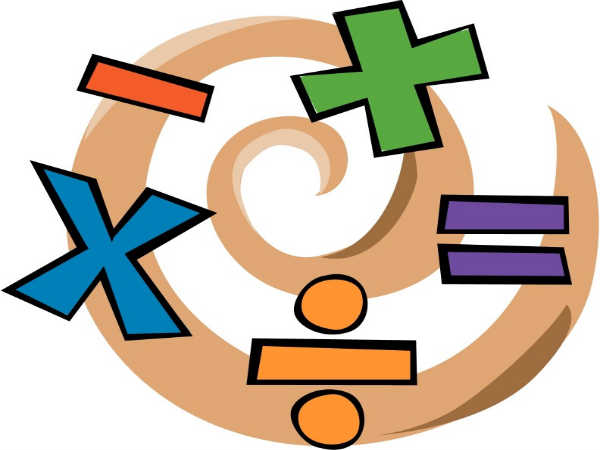# 7 Mathematics Shortcuts That Can Blow You Down

By Malavikka

What comes to your mind when you hear the word mathematics? Numbers? Does that give you a good feeling or an irky feeling? If it's a good feeling, these tricks will enhance your love for math. If it's the irky feeling, we bet you won't feel the same way you do after reading this!

Here are some mathematics shortcuts and hacks that you never knew could make calculations so easy. Read on to get caught in the fascination!

If you want to summon a mind calculator for adding three-digit numbers, here's the trick -

To find the result to this problem, 263 + 726, round up both the numbers and add.

That makes it 270 + 730 = 1000.

Now subtract the difference from the rounded numbers.

270 - 263 = 7, 730 - 726 = 4

Subtract this balance from the sum of the rounded numbers.

That makes it 1000 - 11 = 989.Mersal Mathematics: 5 Shortcuts for Multiplication!

## Subtraction

Did you know how quick it is to subtract a number from 1000? "What is 1000 - 737?" someone might ask. Don't blink, try this hack.

Minus the first two digits from 9 and the last digit from 10.

9 - 7 = 2

9 - 3 = 6

10 - 7 = 3How to Choose the Right Course to Study After Class 12?

## Multiplication

Multiplying any number by 9 is a whale of fun, especially if it is a single-digit number. Just write the number 0 - 9 in ascending and descending order in parallel lines. That's the order of 9 tables! Can't believe it yet? Look for yourself!

0 9

1 8

2 7

3 6

4 5

5 4

6 3

7 2

8 1

9 0E-Learning Trends 2018: 8 Ways Digitisation Makes Studying Addictive

## Division

It's not always multiples of 5 and 10 that are easily divisible numbers. Read on and learn more.

9 - Add all the digits of a number, eg., 466 - add 4 + 6 + 6. The sum is 18. Since 18 is divisible by 9, 466 is divisible by 9, too.

8 - In case the last three digits are evenly divisible by 8 or are 000.

6 - First check if it is an even number, also, the digits when added together should give you a number divisible by 3.

5 - In case the number ends in a 0 or 5.

4 - In case the number ends in 00 or a two-digit number that is evenly divisible by 4.

3 - In case the digits are added together and the output is evenly divisible by 3.

2 - If it ends in 0 or an even digit - 2, 4, 6 or 8.Career Trends 2018: Top 5 Accounting Career Opportunities

## Quick Percentage

What is 30% of 50?

Multiply the numbers 30 x 50 = 1500.

Remove the last two zeros. The answer is 15%.

In case you are wondering what to do when they are not round numbers, round the numbers and multiply to get a rough estimate first. You can work on the accuracy after that.How Can Class 12 Students Start Preparing for Board Exams?

## Approximate Square Roots

It is simple to answer the square root of single digits like 9 or 8 . But what

do you do when asked the square root of 98?

Don't panic. Follow this simple method. You know square of 9 is 81 and the square of 10 is 100. So the answer for square root of 98 must be 9 point something!5 Tips To Be a Successful Working Student

## 11 Magic

Multiplication with 10 is not the only easy times table. Any number multiplied with 11 is equally easy to calculate. Want proof?

Take 43 x 11.

Leave a space between the existing numbers. Say 4_3. Now add up the numbers 4 (4 + 3) 3. The answer is 473. Just in case, the sum makes a two digit, you can carry it over.

Ex: 47 x 11 = 4 (4 + 7) 7.

(4 + 1) 17 = 517.How To Stick To Study Goals and Achieve The Target?

Subscribe Now

--Or--
Select a Field of Study
Select a Course
Select UPSC Exam
Select IBPS Exam
Select Entrance Exam